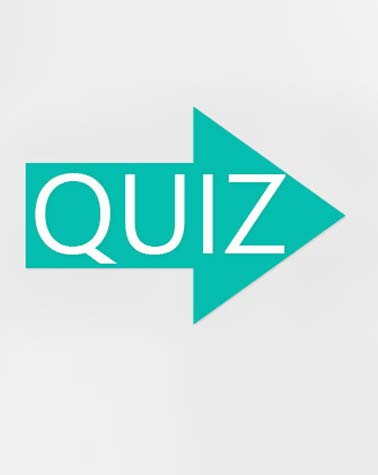# Gimmel Hebrew Letter Lesson 3

10 QuestionsSettings• 1.
What sound does the gimmel make?
• A.

AH

• B.

B

• C.

G

• D.

C

• 2.
What is the numerical value of the gimmel?
• A.

1

• B.

2

• C.

3

• D.

4

• 3.
What sound does this letter make?
• A.

B

• B.

EH

• C.

D

• D.

EE

• 4.
What is the numerical value of this letter?
• A.

1

• B.

2

• C.

3

• D.

4

• 5.
What sound does this make?
• A.

None

• B.

AH

• C.

BA

• D.

EE

• 6.
The dot (dagesh) added to the gimmel changes the sound.
• A.

True

• B.

False

• 7.
What sound does this letter make?
• A.

AH

• B.

BAH

• C.

GAH

• D.

GEE

• 8.
What sound does this letter make?
• A.

GHA

• B.

GEE

• C.

GEH

• D.

GOO

• 9.
• A.

The word is mommy in Hebrew

• B.

The aleph at the beginning is silent and the aleph at the end makes the "EE" sound

• C.

The aleph at the beginning makes the EE sound and the aleph at the end is silent.

• D.

The vowel underneath the second letter makes the AH sound (MAH with the accompanying consonant).

• 10.
This Hebrew word (for father, not daddy) is pronounced "AV".
• A.

True

• B.

False